Home Practice
For learners and parents For teachers and schools
Textbooks
Full catalogue
Pricing SupportLog in

We think you are located in United States. Is this correct?

# Chapter 7: Analytical geometry

## 7.1 Revision (EMCHN)

• Integrate Euclidean Geometry knowledge with Analytical Geometry.
• Emphasize the value and importance of making sketches.
• Emphasize the importance of writing coordinates consistently for the distance formula and gradient.
• Learners must revise the method of completing the square for finding the general form of the equation of a circle with centre $$(a;b)$$.
• Remind learners that the tangent to a circle is perpendicular to the radius (and the diameter).

### Straight line equations (EMCHP)Theorem of Pythagoras: $$AB^2 = AC^2 + BC^2$$ Distance formula: $$AB = \sqrt{(x_2 - x_1)^2 + (y_2 - y_1)^2}$$ Gradient: $$m_{AB} = \frac{y_2 - y_1}{x_2 - x_1} \quad \text{ or } \quad m_{AB} = \frac{y_1 - y_2}{x_1 - x_2}$$ Mid-point of a line segment: $$M(x;y) = \left( \frac{x_1 + x_2}{2}; \frac{y_1 + y_2}{2} \right)$$ Points on a straight line: $$m_{AB} = m_{AM} = m_{MB}$$
 Horizontal lines: $$y = k$$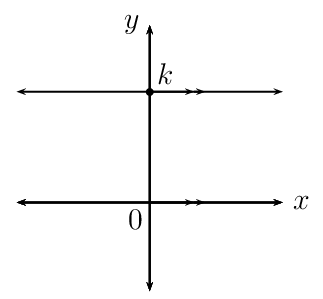Vertical lines: $$x = k$$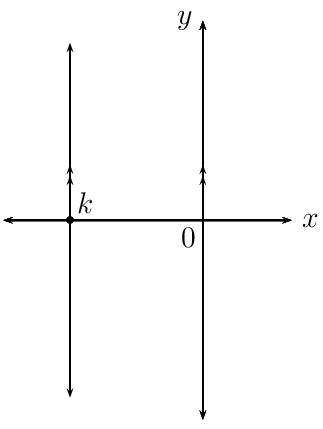Two-point form: $$\dfrac{y - y_1}{x - x_1} = \dfrac{y_2 - y_1}{x_2 - x_1}$$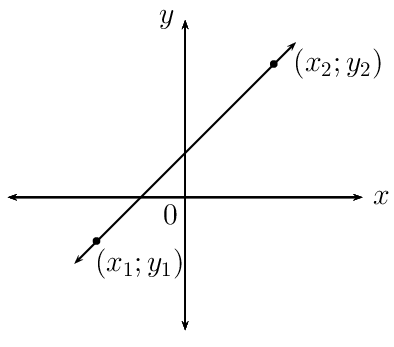Gradient-point form: $$y - y_1 = m (x - x_{1})$$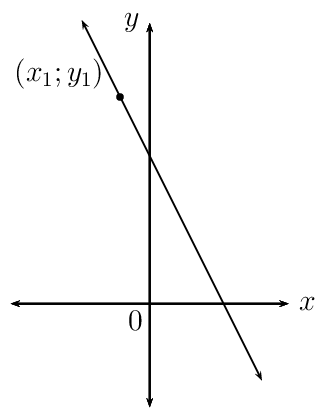Gradient-intercept form: $$y = mx + c$$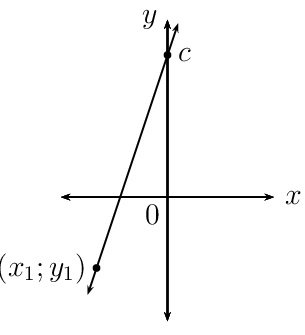## Worked example 1: Revision

Given quadrilateral $$PQRS$$ with vertices $$P(0;3), Q(4;3), R(5;-1)$$ and $$S(1;-1)$$.

1. Determine the equation of the lines $$PS$$ and $$QR$$.
2. Show that $$PS \parallel QR$$.
3. Calculate the lengths of $$PS$$ and $$QR$$.
4. Determine the equation of the diagonal $$QS$$.
5. What type of quadrilateral is $$PQRS$$?

### Draw a sketch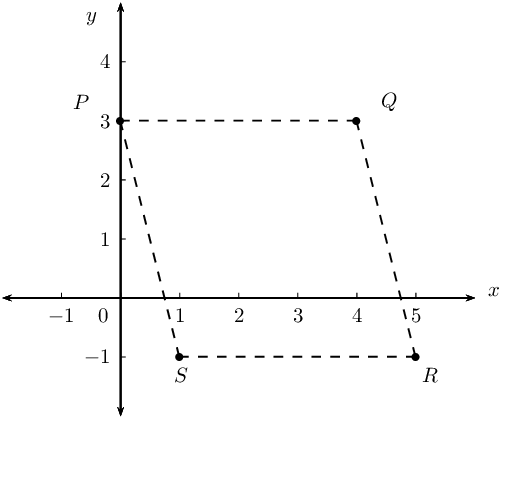### Use the given information to determine the equation of lines $$PS$$ and $$QR$$

\begin{align*} \text{Gradient: } & m = \frac{y_2 - y_1}{x_2 - x_1} \\ & \\ \text{Two-point form: } & \dfrac{y - y_1}{x - x_1} = \dfrac{y_2 - y_1}{x_2 - x_1} \\ & \\ \text{Gradient-intercept form: } & y = mx + c \end{align*}

Determine the equation of the line $$PS$$ using the two point form of the straight line equation:

$x_1 = 0; \qquad y_1 = 3; \qquad x_2 = 1; \qquad y_2 = -1$ \begin{align*} \dfrac{y - y_1}{x - x_1} &= \dfrac{y_2 - y_1}{x_2 - x_1} \\ \dfrac{y - 3}{x - 0} &= \dfrac{-1 - 3}{1 - 0} \\ \dfrac{y - 3}{x} &= -4 \\ y - 3 &= -4x \\ \therefore y &= -4x + 3 \end{align*}

Determine the equation of the line $$QR$$ using the gradient-intercept form of the straight line equation:

\begin{align*} m_{QR} &= \frac{y_2 - y_1}{x_2 - x_1} \\ &= \frac{-1-3}{5-4} \\ &= \frac{-4}{1} \\ &= -4 \\ y &= mx + c \\ y &= -4x + c \\ \text{Substitute } (4;3) \quad 3 &= -4(4) + c \\ \therefore c &= 19 \\ y &= -4x + 19 \end{align*}

There is often more than one method for determining the equation of a line. The different forms of the straight line equation are used, depending on the information provided in the problem.

### Show that line $$PS$$ and line $$QR$$ have equal gradients

\begin{align*} y &= -4x + 3 \\ \therefore m_{PS} &= -4 \\ \text{And } y &= -4x + 19 \\ \therefore m_{QR} &= -4 \\ \therefore m_{PS} &= m_{QR} \\ \therefore PS &\parallel QR \end{align*}

### Use the distance formula to determine the lengths of $$PS$$ and $$QR$$

\begin{align*} PS &= \sqrt{ \left( x_2 - x_1 \right)^2 + \left( y_2 - y_1 \right)^2} \\ &= \sqrt{ \left(1-0 \right)^2 + \left( -1-3 \right)^2} \\ &= \sqrt{ 1 + \left( -4 \right)^2} \\ &= \sqrt{17} \text{ units} \end{align*}\begin{align*} QR &= \sqrt{ \left( x_2 - x_1 \right)^2 + \left( y_2 - y_1 \right)^2} \\ &= \sqrt{ \left( 5-4 \right)^2 + \left( -1-3 \right)^2} \\ &= \sqrt{ 1 + \left( -4 \right)^2} \\ &= \sqrt{17} \text{ units} \end{align*}

### Determine the equation of the diagonal $$QS$$

Determine the gradient of the line:

\begin{align*} m_{QS} &= \frac{y_2 - y_1}{x_2 - x_1} \\ &= \frac{-1 -3}{1-4} \\ &= \frac{-4}{-3} \\ &= \frac{4}{3} \end{align*}

Use gradient and the point $$Q(4;3)$$ to determine the equation of the line $$QS$$:

\begin{align*} y - y_{1}&= m(x - x_{1}) \\ y - y_{1}&= \frac{4}{3}(x - x_{1}) \\ \text{Substitute } (4;3) \quad y - 3 &= \frac{4}{3}(x - 4) \\ y - 3 &= \frac{4}{3}x - \frac{16}{3} \\ y &= \frac{4}{3}x - \frac{16}{3} + 3 \\ \therefore y &= \frac{4}{3}x - \frac{7}{3} \end{align*}

### Examine the properties of quadrilateral $$PQRS$$

We have shown that $$PS \parallel QR$$ and $$PS = QR$$, therefore quadrilateral $$PQRS$$ is a parallelogram (one pair of opposite sides equal and parallel).

## Revision

Textbook Exercise 7.1

Determine the following for the line segment between the given points:

• length
• mid-point
• equation

$$(-2;-4)$$ and $$(3;11)$$

\begin{align*} \text{Distance } &= \sqrt{(x_{2} - x_{1})^{2} + (y_{2} - y_{1})^2} \\ &= \sqrt{(3 - (-2))^{2} + (11 - (-4))^2} \\ &= \sqrt{(5)^{2} + (15)^2} \\ &= \sqrt{25 + 225} \\ &= \sqrt{250} \\ &= 5\sqrt{10} \end{align*} \begin{align*} M(x;y) &= \left( \frac{x_{1} + x_{2}}{2}; \frac{y_{1} + y_{2}}{2} \right) \\ &= \left( \frac{-2 + 3}{2}; \frac{-4 + 11 }{2} \right) \\ &= \left( \frac{1}{2}; \frac{7}{2} \right) \end{align*} \begin{align*} m &= \frac{y_{2} - y_{1}}{x_{2}- x_{1}} \\ &= \frac{11 - (-4)}{3 - (-2)} \\ &= \frac{15}{5} \\ &= 3 \end{align*} \begin{align*} y &= mx + c \\ y &= 3x + c \\ \text{Substitute } (-2;-4) \quad -4 &= 3(-2) + c \\ -4 + 6 &= c \\ 2 &= c \\ \therefore y &= 3x + 2 \end{align*}

$$(-5;-3)$$ and $$(10;6)$$

\begin{align*} \text{Distance } &= \sqrt{(x_{2} - x_{1})^{2} + (y_{2} - y_{1})^2} \\ &= \sqrt{(10 - (-5))^{2} + (6 - (-3))^2} \\ &= \sqrt{(15)^{2} + (9)^2} \\ &= \sqrt{225+81} \\ &= \sqrt{306} \end{align*} \begin{align*} M(x;y) &= \left( \frac{x_{1} + x_{2}}{2}; \frac{y_{1} + y_{2}}{2} \right) \\ &= \left( \frac{-5 + 10}{2}; \frac{-3 + 6 }{2} \right) \\ &= \left( \frac{5}{2}; \frac{3}{2} \right) \end{align*} \begin{align*} m &= \frac{y_{2} - y_{1}}{x_{2}- x_{1}} \\ &= \frac{6 - (-3)}{10 - (-5)} \\ &= \frac{9}{15} \\ &= \frac{3}{5} \end{align*} \begin{align*} y &= mx + c \\ y &= \frac{3}{5} x + c \\ \text{Substitute } (10;6) \quad 6 &= \frac{3}{5} (10) + c \\ 6 - 6 &= c \\ 0 &= c \\ \therefore y &= \frac{3}{5}x \end{align*}

$$(h; -h - k)$$ and $$(2k; h - 5k)$$

\begin{align*} \text{Distance } &= \sqrt{(x_{2} - x_{1})^{2} + (y_{2} - y_{1})^2} \\ &= \sqrt{(h - 2k)^{2} + (-h - k - (h - 5k))^2} \\ &= \sqrt{(h - 2k)^{2} + (-2h + 4k)^2} \\ &= \sqrt{h^2 - 4hk + 4k^2 + +4h^2 -16hk + 16k^2} \\ &= \sqrt{5h^2 - 20hk + 20k^2} \end{align*} \begin{align*} M(x;y) &= \left( \frac{x_{1} + x_{2}}{2}; \frac{y_{1} + y_{2}}{2} \right) \\ &= \left( \frac{h + 2k}{2}; \frac{-h - k + (h - 5k) }{2} \right) \\ &= \left( \frac{h + 2k}{2}; \frac{-6k) }{2} \right) \\ &= \left( \frac{h + 2k}{2}; - 3k \right) \end{align*} \begin{align*} m &= \frac{y_{2} - y_{1}}{x_{2}- x_{1}} \\ &= \frac{(h - 5k) - (-h - k)}{2k - h} \\ &= \frac{2h - 4k}{-h + 2k} \\ &= \frac{-2(-h + 2k)}{-h + 2k} \\ &= -2 \end{align*} \begin{align*} y &= mx + c \\ y &= -2x + c \\ \text{Substitute } (h; -h-k) \quad -h - k &= -2(h) + c \\ -h + 2h - k &= c \\ h - k &= c \\ \therefore y &= -2x + h - k \end{align*}

$$(2;9)$$ and $$(0;-1)$$

\begin{align*} \text{Distance } &= \sqrt{(x_{2} - x_{1})^{2} + (y_{2} - y_{1})^2} \\ &= \sqrt{(2 - (0))^{2} + (9 - (-1))^2} \\ &= \sqrt{(2)^{2} + (10)^2} \\ &= \sqrt{4 + 100} \\ &= \sqrt{104} \end{align*} \begin{align*} M(x;y) &= \left( \frac{x_{1} + x_{2}}{2}; \frac{y_{1} + y_{2}}{2} \right) \\ &= \left( \frac{2 + 0}{2}; \frac{9 - 1 }{2} \right) \\ &= \left( 1 ; 4 \right) \end{align*} \begin{align*} m &= \frac{y_{2} - y_{1}}{x_{2}- x_{1}} \\ &= \frac{-1 - 9}{0 - 2} \\ &= \frac{-10}{-2} \\ &= 5 \end{align*} \begin{align*} y &= mx + c \\ y &= 5 x + c \\ \text{Substitute } (0;-1) \quad -1 &= 5(0) + c \\ -1 &= c \\ \therefore y &= 5x - 1 \end{align*}

The line joining $$A(x;y)$$ and $$B(-3;6)$$ has the mid-point $$M(2;3)$$. Determine the values of $$x$$ and $$y$$.

\begin{align*} M(x;y) &= \left( \frac{x_{A} + x_{B}}{2}; \frac{y_{A} + y_{B}}{2} \right) \\ M(2;3) &= \left( \frac{x - 3}{2}; \frac{y + 6}{2} \right) \\ \therefore 2 &= \frac{x - 3}{2} \\ 4 &= x - 3 \\ \therefore 7 &= x \\ \text{And } 3 &= \frac{y + 6}{2} \\ 6 &= y + 6 \\ \therefore 0 &= y \end{align*} $$A(7;0)$$

Given $$F(2; 11), G(-4; r)$$ and length $$FG = 6\sqrt{5}$$ units, determine the value(s) of $$r$$.

There are two possible values of $$r$$ such that the length $$FG = 6\sqrt{5}$$ units: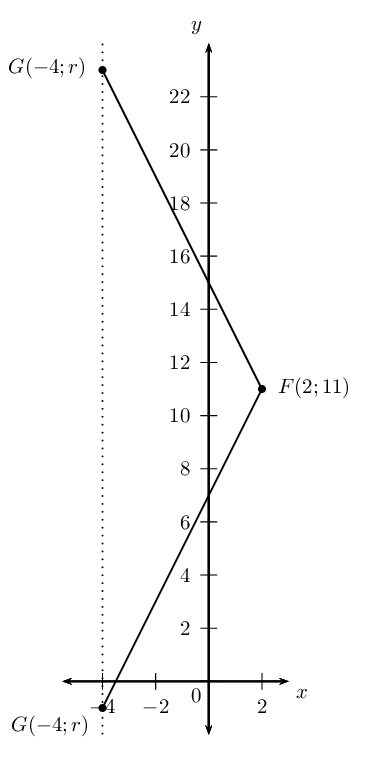Determine the equation of the straight line:

passing through the point $$(\frac{1}{2};4)$$ and $$(1;5)$$.

\begin{align*} \frac{y - y_{1}}{x - x_{2}} &= \frac{y_{2} - y_{1}}{x_{2} - x_{1}} \\ \frac{y - 4}{x - \frac{1}{2}} &= \frac{5 - 4}{1 - \frac{1}{2}} \\ \frac{y - 4}{x - \frac{1}{2}} &= \frac{1}{\frac{1}{2}} \\ \frac{y - 4}{x - \frac{1}{2}} &= 2 \\ y - 4 &= 2 \left(x - \frac{1}{2} \right) \\ y &= 2x - 1 + 4 \\ \therefore y &= 2x + 3 \end{align*}

passing through the points $$(2;-3)$$ and $$(-1;0)$$.

\begin{align*} y &= mx + c \\ -3 &= 2m + c \ldots (1) \\ 0 &= -m + c \ldots (2) \\ (1) - (2): \quad -3 &= 2m + m \\ -3 &= 3m \\ \therefore -1 &= m \\ \therefore c &= -1 \\ \therefore y &= -x - 1 \end{align*}

passing through the point $$(9;1)$$ and with $$m=\frac{1}{3}$$.

\begin{align*} y - y_1 &= m(x - x_1)\\ y - 1 &= \frac{1}{3}(x - 9)\\ y - 1 &= \frac{1}{3}x - 3 \\ \therefore y &= \frac{1}{3}x -2 \end{align*}

parallel to the $$x$$-axis and passing through the point $$(0;-4)$$.

\begin{align*} y - y_1 &= m(x - x_1) \\ y -(-4)&= 0(x - 0) \\ \therefore y &= -4 \end{align*}

passing through the point $$(\frac{1}{2};-1)$$ and with $$m=-4$$.

\begin{align*} y - y_1 &= m(x - x_1) \\ y - (-1) &= -4(x - \frac{1}{2}) \\ y + 1 &=-4x + 2 \\ \therefore y &= -4x + 1 \end{align*}

perpendicular to the $$x$$-axis and passing through the point $$(5;0)$$.

$$x = 5$$

with undefined gradient and passing through the point $$(\frac{3}{4};0)$$.

$$x = \frac{3}{4}$$

with $$m= 2p$$ and passing through the point $$(3; 6p + 3)$$.

\begin{align*} y - y_1 &= m(x - x_1) \\ y - (6p + 3) &= 2p(x - 3 ) \\ y - 6p - 3 &= 2px - 6p \\ \therefore y &= 2px + 3 \end{align*}

which cuts the $$y$$-axis at $$y=-\frac{3}{5}$$ and with $$m=4$$.

\begin{align*} y &= mx + c \\ y &= mx - \frac{3}{5} \\ \therefore y &= 4x -\frac{3}{5} \end{align*}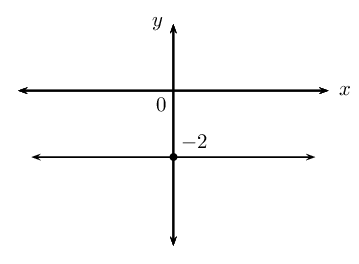$$y = - 2$$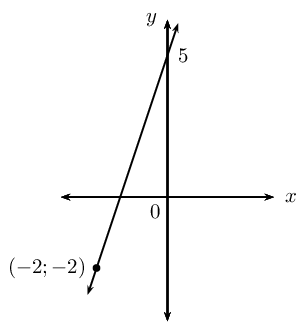\begin{align*} c &= 5 \\ y &= mx + c \\ y &= mx + 5 \\ \text{Substitute } (-2;-2) \quad -2 &= -2m + 5 \\ -7 &= -2m \\ m &= \frac{7}{2} \\ \therefore y &= \frac{7}{2}x + 5 \end{align*}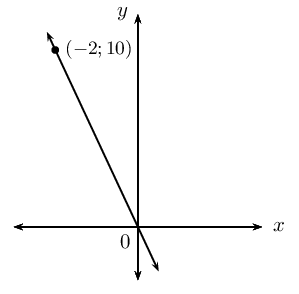\begin{align*} c &= 0 \\ y &= mx + c \\ y &= mx + 0\\ \text{Substitute } (-2;10) \quad 10 &= -2m \\ -2m &= 10 \\ \therefore m &= -5 \\ \therefore y &= -5x \end{align*}

### Inclination of a line (EMCHQ)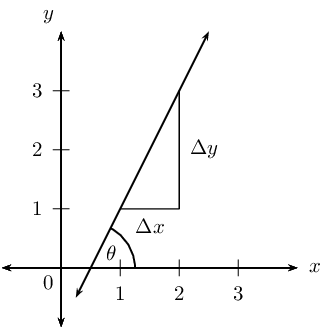The diagram shows a straight line which forms an acute angle $$\theta$$ with the positive $$x$$-axis. This is called the angle of inclination of a straight line.

The gradient of a straight line is equal to the tangent of the angle formed between the line and the positive direction of the $$x$$-axis.

$m = \tan \theta \qquad \text{ for } \text{0}° \leq \theta < \text{180}°$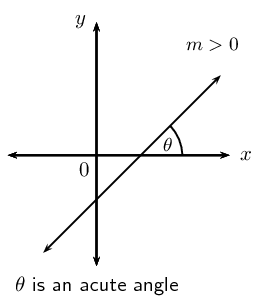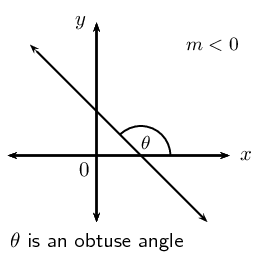A line with a positive gradient ($$m > 0$$) has an acute angle of inclination ($$\text{0}° < \theta < \text{90}°$$).

For example, we can determine the angle of inclination of a line with $$m = \text{1,2}$$:

\begin{align*} \tan \theta &= m \\ &= \text{1,2} \\ \therefore \theta &= \tan^{-1}(\text{1,2}) \\ &= \text{50,2}° \end{align*}

If we are calculating the angle of inclination of a line with a negative gradient ($$m < 0$$), then we add $$\text{180}°$$ to change the negative angle to an obtuse angle ($$\text{90}° < \theta < \text{180}°$$).

For example, we can determine the angle of inclination for a line with $$m = -\text{0,7}$$:

\begin{align*} \tan \theta &= m \\ &= -\text{0,7} \\ \therefore \theta &= \tan^{-1}(-\text{0,7}) \\ &= -\text{35,0}° \\ \text{Obtuse angle: } \theta &= -\text{35,0}° + \text{180}° \\ &= \text{145}° \end{align*}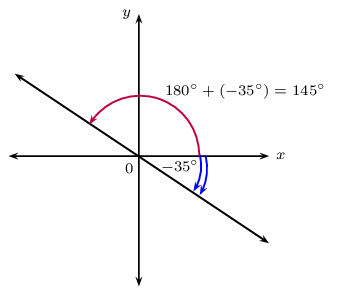## Worked example 2: Inclination of a straight line

Determine the acute angle (correct to $$\text{1}$$ decimal place) between the line passing through the points $$P(-2;0)$$ and $$Q(3;1)$$ and the straight line $$y = - \frac{4}{3}x + 5$$.

### Draw a sketch

Draw the line through points $$P(-2;0)$$ and $$Q(3;1)$$ and the line $$y = - \frac{4}{3}x + 5$$ on a suitable system of axes. Label $$\alpha$$ and $$\beta$$, the angles of inclination of the two lines. Label $$\theta$$, the acute angle between the two straight lines.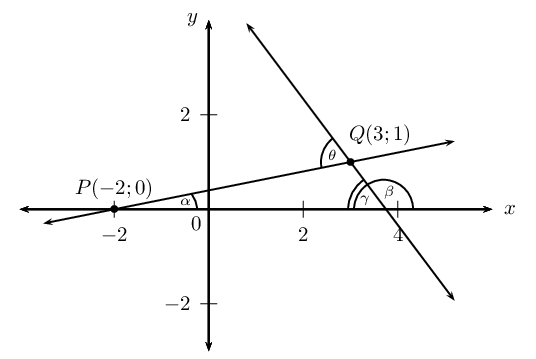Notice that $$\alpha$$ and $$\theta$$ are acute angles and $$\beta$$ is an obtuse angle.

$\begin{array}{rll} \gamma &= \text{180}° - \beta & (\angle \text{ on str. line}) \\ \text{and } \theta &= \alpha + \gamma \quad & (\text{ext. } \angle \text{ of } \triangle = \text{ sum int. opp}) \\ \therefore \theta &= \alpha + (\text{180}° - \beta) \\ &= \text{180}° + \alpha - \beta \end{array}$

### Use the gradient to determine the angle of inclination $$\beta$$

From the equation $$y = - \frac{4}{3}x + 5$$ we see that $$m < 0$$, therefore $$\beta$$ is an obtuse angle.

\begin{align*} m &= -\frac{4}{3} \\ \tan \beta &= -\frac{4}{3} \\ \therefore \beta &= \tan^{-1} \left(-\frac{4}{3}\right) \\ &= -\text{53,1}° \\ \beta &= -\text{53,1}° + \text{180}°\\ &= \text{126,9}° \end{align*}

### Determine the gradient and angle of inclination of the line through $$P$$ and $$Q$$

Determine the gradient \begin{align*} m & = \frac{y_P - y_Q}{x_P - x_Q} \\ & = \dfrac{- 1}{-5} \\ &= \frac{1}{5} \end{align*}

Determine the angle of inclination \begin{align*} \tan \alpha & = m\\ & = \frac{1}{5} \\ \therefore \alpha & = \tan^{-1} \left( \frac{1}{5} \right) \\ &= \text{11,3}° \end{align*}

\begin{align*} \theta & = \text{180}° + \alpha - \beta \\ & = \text{180}° + \text{11,3}° - \text{126,9}° \\ & = \text{64,4}° \end{align*}

The acute angle between the two straight lines is $$\text{64,4}$$°.

### Parallel and perpendicular lines (EMCHR)

 Parallel lines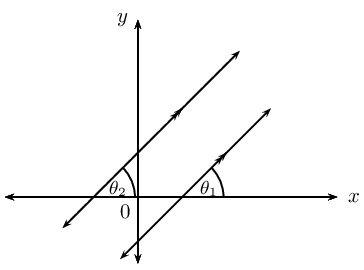$$m_1 = m_2$$ $$\theta_1 = \theta_2$$ Perpendicular lines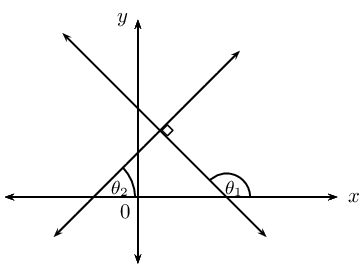$$m_1 \times m_2 = -1$$ $$\theta_{1} = \text{90}° + \theta_{2}$$

## Worked example 3: Parallel lines

Line $$AB$$ passes through the point $$A(0;3)$$ and has an angle of inclination of $$\text{153,4}$$°.

1. Determine the equation of line $$CD$$ which passes through the point $$C(2;-3)$$ and is parallel to $$AB$$.
2. Determine the equation of line $$EF$$, which passes through the origin and is perpendicular to both $$AB$$ and $$CD$$.
3. Sketch lines $$AB$$, $$CD$$ and $$EF$$ on the same system of axes.
4. Use two different methods to determine the angle of inclination of $$EF$$.

### Draw a rough sketch and use the angle of inclination to determine the equation of $$CD$$Since we are given $$AB \parallel CD$$,

\begin{align*} m_{CD} = m_{AB} &= -\text{0,5} = -\frac{1}{2} \\ y - y_1 &= m(x - x_1) \\ y - y_1 &= -\frac{1}{2}(x - x_1) \end{align*}

Substitute the given point $$(2;-3)$$:

\begin{align*} y - (-3) & = -\frac{1}{2}(x - 2) \\ y + 3 & = -\frac{1}{2}x + 1\\ y & = -\frac{1}{2}x - 2 \end{align*}

### Determine the equation of $$EF$$

$$EF$$ is perpendicular to $$AB$$, therefore the product of their gradients is equal to $$-1$$:

\begin{align*} m_{AB} \times m_{EF} & = -1 \\ -\frac{1}{2} \times m_{EF} & = - 1 \\ \therefore m_{EF} & = 2 \end{align*}

We know line $$EF$$ passes through $$(0;0)$$, therefore the equation of the line is:

$y = 2x$

### Determine the angle of inclination of $$EF$$

Let the angle of inclination of $$EF$$ be $$\beta$$.

Method $$\text{1}$$:

\begin{align*} \beta & = \text{153,4}° - \text{90}° \\ & = \text{63,4}° \end{align*}

Method $$\text{2}$$:

\begin{align*} m & = 2 \\ \tan \beta & = 2 \\ \therefore \beta & = \text{63,4}° \end{align*}

### Draw a sketch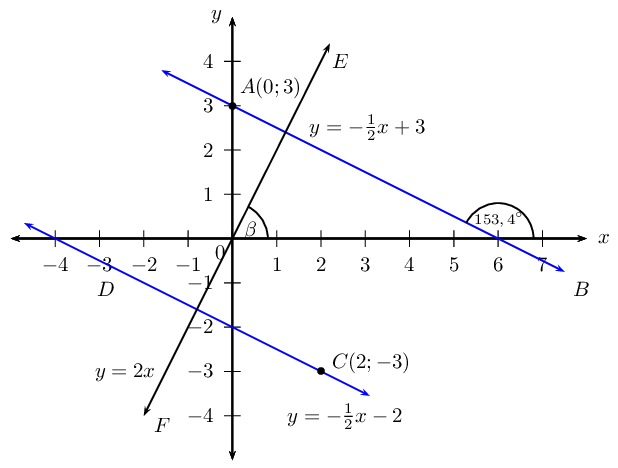## Inclination of a straight line

Textbook Exercise 7.2

Determine the angle of inclination (correct to $$\text{1}$$ decimal place) for each of the following:

a line with $$m = \frac{3}{4}$$

\begin{align*} \tan \theta &= m \\ &= \frac{3}{4} \\ \theta &= \tan^{-1} \left( \text{0,75} \right) \\ \therefore \theta &= \text{36,9}° \end{align*}

$$6 + x = 2y$$

\begin{align*} 6 + x &= 2y \\ 2y &= x + 6 \\ y &= \frac{1}{2}x + 3 \\ \tan \theta &= m \\ &= \frac{1}{2} \\ \theta &= \tan^{-1} \left( \text{0,5} \right) \\ \therefore \theta &= \text{26,6}° \end{align*}

the line passes through the points $$(-4;0)$$ and $$(2;6)$$

\begin{align*} m &= \frac{y_2 - y_1}{x_2 - x_1} \\ &= \frac{6 - 0}{2 - (-4)} \\ &= \frac{6}{6} \\ \therefore m &= 1 \\ \tan \theta &= 1 \\ \theta &= \tan^{-1} \left( \text{1} \right) \\ \therefore \theta &= \text{45}° \end{align*}

$$y=4$$

Horizontal line

a line with a gradient of $$\text{1,733}$$

\begin{align*} m &= \text{1,733} \\ \theta &= \tan^{-1} \left( \text{1,733} \right) \\ \therefore \theta &= \text{60}° \end{align*}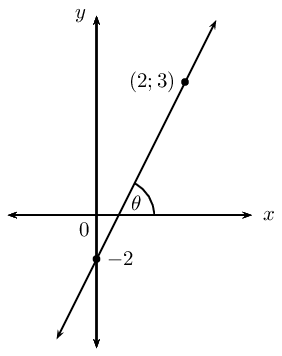\begin{align*} m &= \frac{y_2 -y_1}{x_2 - x_1} \\ &= \frac{3 + 2}{2 - 0} \\ &= \frac{5}{2} \\ \theta &= \tan^{-1} \left( \frac{5}{2} \right) \\ \therefore \theta &= \text{68,2}° \end{align*}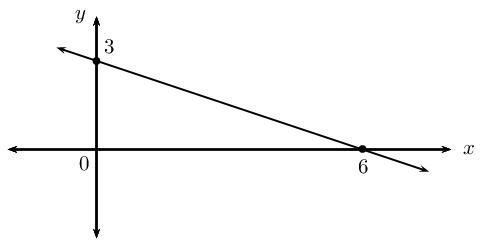\begin{align*} m &= \frac{y_2 -y_1}{x_2 - x_1} \\ &= \frac{3 - 0}{0 - 6} \\ &= \frac{3}{-6} \\ \therefore m &= -\frac{1}{2} \\ \theta &= \tan^{-1} \left( -\frac{1}{2} \right) \\ \therefore \theta &= -\text{26,6}° \\ \therefore \theta &= \text{180} ° - \text{26,6} ° \\ \therefore \theta &= \text{153,4} ° \end{align*}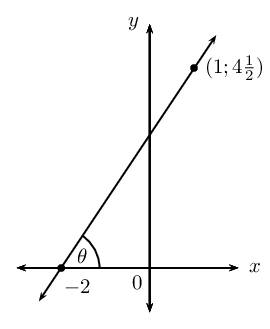\begin{align*} m &= \frac{y_2 -y_1}{x_2 - x_1} \\ &= \frac{\frac{9}{2} - 0}{1 + 2} \\ &= \frac{\frac{9}{2}}{3} \\ &= \frac{3}{2} \\ \theta &= \tan^{-1} \left( \frac{3}{2} \right) \\ \therefore \theta &= \text{56,3}° \end{align*}

Find the angle between the line $$2y = 5x$$ and the line passing through points $$T(2;1\frac{1}{3})$$ and V$$(-3;3)$$.

Let the angle of inclination for the line $$2y = 5x$$ be $$\beta$$ and let the angle of inclination for the other line be $$\alpha$$. Let the angle between the two lines be $$\theta$$.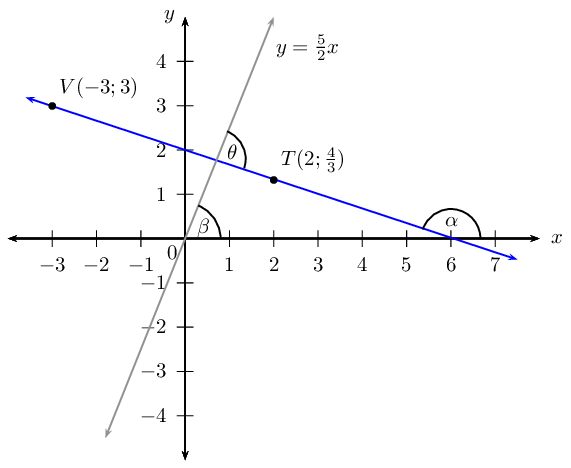Determine the equation of the straight line that passes through the point $$(1;2)$$ and is parallel to the line $$y + 3x = 1$$.

\begin{align*} y + 3x &= 1 \\ y &= -3x + 1 \\ \therefore m &= -3 \\ y - y_1&= m(x - x_1) \\ y - 2 &= -3(x - 1) \\ y &= -3x + 3 + 2 \\ \therefore y &= -3x + 5 \end{align*}

Determine the equation of the straight line that passes through the point $$(-4;-4)$$ and is parallel to the line with angle of inclination $$\theta = \text{56,31}°$$.

\begin{align*} \theta &= \text{56,31}° \\ \therefore m &= \tan \theta \\ &= \tan \text{56,31}° \\ \therefore m &= \text{1,5} \\ y - y_1&= m(x - x_1) \\ y + 4 &= \frac{3}{2}(x + 4) \\ y &= \frac{3}{2}x + 6 - 4 \\ \therefore y &= \frac{3}{2}x + 2 \end{align*}

Determine the equation of the straight line that passes through the point $$(1;-6)$$ and is perpendicular to the line $$5y = x$$.

\begin{align*} 5y &= x \\ y &= \frac{1}{5}x \\ \therefore m_1 &= \frac{1}{5} \\ \text{For } \perp: m_1 \times m_2 &= -1 \\ \frac{1}{5} \times m_2 &= -1 \\ \therefore m_2 &= -5 \\ y &= mx + c \\ y &= -5x + c \\ \text{Substitute } (1;-6): \quad -6 &= -5(1) + c \\ -6 &= -5 + c \\ \therefore c &=-1 \\ \therefore y &= -5x - 1 \end{align*}

Determine the equation of the straight line that passes through the point $$(3;-1)$$ and is perpendicular to the line with angle of inclination $$\theta = \text{135}°$$.

\begin{align*} \theta &= \text{135}° \\ \therefore m_{1} &= \tan \theta \\ &= \tan \text{135}° \\ \therefore m_{1} &= -1 \\ m_{1} \times m_{2} &= -1 \\ \therefore m_{2} &= 1 \\ y &= mx + c \\ y &= x + c \\ \text{Substitute } (3;-1): \quad -1 &= (3) + c \\ \therefore c &= -4 \\ \therefore y &= x - 4 \end{align*}

$$A(2;3)$$, $$B(-4;0)$$ and $$C(5;-3)$$ are the vertices of $$\triangle ABC$$ in the Cartesian plane. $$AC$$ intersects the $$x$$-axis at $$D$$. Draw a sketch and determine the following:

the equation of line $$AC$$

Draw a sketch: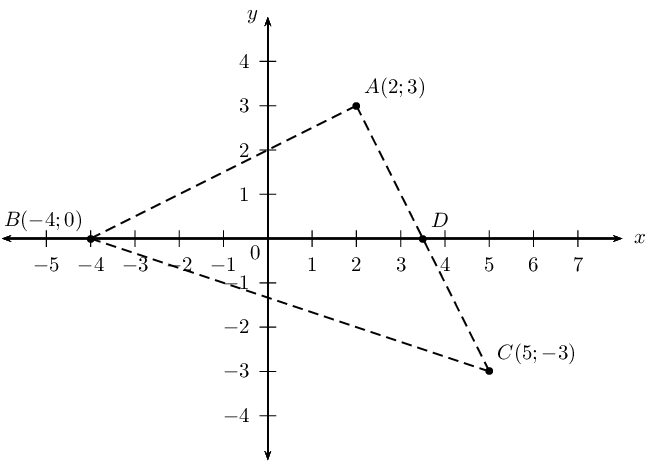the coordinates of point $$D$$

\begin{align*} y &= -2x + 7 \\ 0 &= -2x + 7 \\ \therefore x &= \frac{7}{2} \\ \therefore &D\left( \frac{7}{2}; 0 \right) \end{align*}

the angle of inclination of $$AC$$

\begin{align*} \therefore m &= -2 \\ \tan \theta &= m \\ \tan \theta &= -2 \\ \therefore \theta &= \tan^{-1} \left( -2 \right) \\ \theta &= -\text{63,4} ° + \text{180} ° \\ \therefore \theta &= \text{116,6} ° \end{align*}

the gradient of line $$AB$$

\begin{align*} m &= \frac{y_2 - y_1}{x_2 - x_1}\\ &= \frac{3-0}{2+4} \\ &= \frac{3}{6} \\ \therefore m &= \frac{1}{2} \end{align*}

$$B\hat{A}C$$

$$B\hat{A}C = \text{90} °$$ because $$m_{AB} \times m_{AC} = -1$$

the equation of the line perpendicular to $$AB$$ and passing through the origin

\begin{align*} m_{PQ} &= \frac{1}{2}\\ \therefore m_{\perp}&= -2 \\ y &= -2x + c \\ c &= 0 \\ \therefore y &= -2x \end{align*}

the mid-point $$M$$ of $$BC$$

\begin{align*} M(x;y) &= \left( \frac{x_1 + x_2}{2}; \frac{y_1 + y_2}{2} \right) \\ &= \left( \frac{-4+5}{2}; \frac{0-3}{2} \right) \\ &= \left( \frac{1}{2}; -\frac{3}{2} \right) \end{align*}

the equation of the line parallel to $$AC$$ and passing through point $$M$$

\begin{align*} m &= -2 \\ y &= mx + c \\ y &= -2x + c \\ \text{Substitute } \left( \frac{1}{2}; -\frac{3}{2} \right): \quad -\frac{3}{2} &= -2\left( \frac{1}{2} \right) + c \\ c &= -\frac{1}{2} \\ y &= - 2x -\frac{1}{2} \end{align*}

Points $$F(-3;5)$$, $$G(-7;-4)$$ and $$H(2;0)$$ are given.

Plot the points on the Cartesian plane.Determine the coordinates of $$I$$ if $$FGHI$$ is a parallelogram.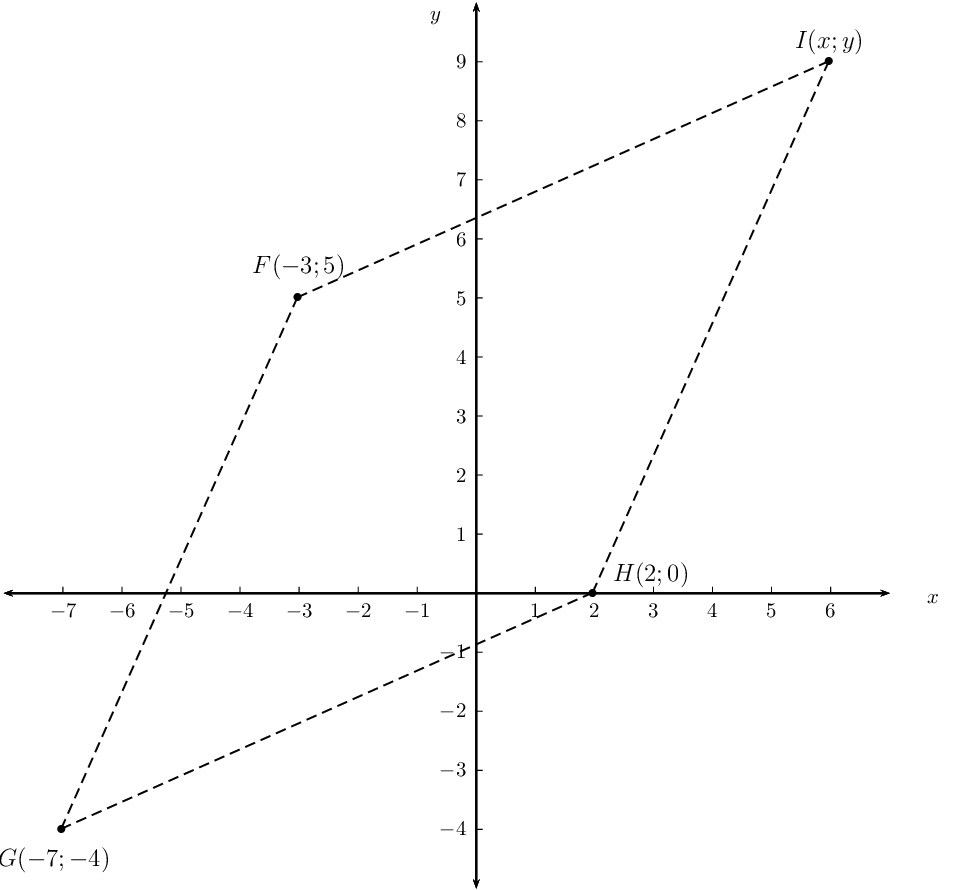Prove that $$FGHI$$ is a rhombus.

\begin{align*} FG &= \sqrt{(x_2 - x_1)^2 + (y_2 - y_1)^2} \\ &= \sqrt{(-7-(-3))^2 + (-4-5)^2} \\ &= \sqrt{(-4)^2 + (-9)^2} \\ &= \sqrt{16 + 81} \\ &= \sqrt{97} \\ HG &= \sqrt{(x_2 - x_1)^2 + (y_2 - y_1)^2} \\ &= \sqrt{(2+7)^2 + (0+4)^2} \\ &= \sqrt{(9)^2 + (4)^2} \\ &= \sqrt{81 + 16} \\ &= \sqrt{97} \\ \therefore FG &= HG \\ \therefore FGHI &\text{ is a rhombus (parallelogram with adj. sides equal ) } \end{align*}

Given points $$S(2;5)$$, $$T(-3;-4)$$ and $$V(4;-2)$$.

Show that the equation of the line $$ST$$ is $$5y = 9x + 7$$.

Draw a sketch: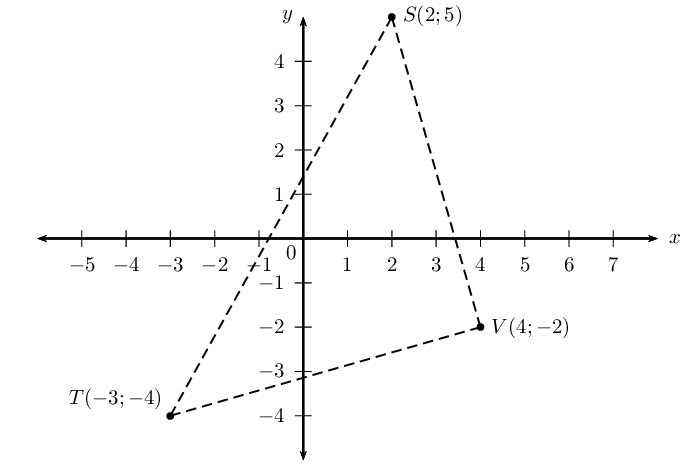Determine the size of $$T\hat{S}V$$.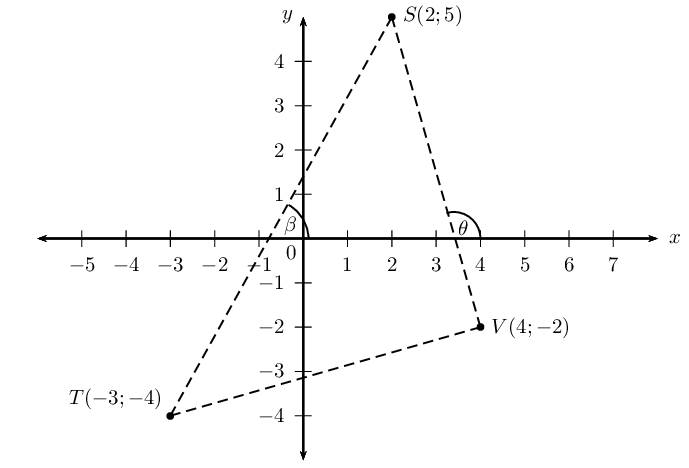Let the angle of inclination of the line $$ST$$ be $$\beta$$.

Let the angle of inclination of the line $$SV$$ be $$\theta$$.

$$T\hat{S}V = \theta - \beta.$$ (exterior $$\angle$$ of $$\triangle =$$ sum on opp. interior $$\angle$$'s)

\begin{align*} m_{ST} &= \frac{9}{5} \\ \tan \beta &= \frac{9}{5} \\ \therefore \beta &= \tan^{-1} \left( \frac{9}{5} \right) \\ &= \text{60,9} ° \\ m_{SV} &= \frac{5+2}{2-4} \\ &= \frac{7}{-2} \\ \tan \theta &= -\frac{7}{2} \\ \therefore \theta &= \tan^{-1} \left( -\frac{7}{2} \right) \\ &= -\text{74,1} ° + \text{180} ° \\ &= \text{105,9} ° \\ \therefore T\hat{S}V &= \text{105,9} ° - \text{60,9} ° \\ &= \text{45} ° \end{align*}